Courses

# Test: Mensuration- 5

## 15 Questions MCQ Test Quantitative Aptitude (Quant) | Test: Mensuration- 5

Description
This mock test of Test: Mensuration- 5 for Quant helps you for every Quant entrance exam. This contains 15 Multiple Choice Questions for Quant Test: Mensuration- 5 (mcq) to study with solutions a complete question bank. The solved questions answers in this Test: Mensuration- 5 quiz give you a good mix of easy questions and tough questions. Quant students definitely take this Test: Mensuration- 5 exercise for a better result in the exam. You can find other Test: Mensuration- 5 extra questions, long questions & short questions for Quant on EduRev as well by searching above.
QUESTION: 1

### The radii of two spheres are in the ratio of 1 : 2. Find the ratio of their surface areas.

Solution: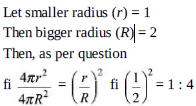QUESTION: 2

### Find the number of bricks, each measuring 25 cm × 12.5 cm × 7.5 cm, required to construct a wall 12 m long, 5 m high and 0.25 m thick, while the sand and cement mixture occupies 5% of the totalvolume of wall.

Solution:

Volume of wall = 1200 × 500 × 25 = 15000000 cm3
Volume of cement = 5% of 15000000 = 750000 cm3
Remaining volume = 15000000 – 750000 = 14250000 cm3
Volume of a brick = 25 × 12.5 × 7.5 = 2343.75 cm3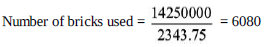QUESTION: 3

### In a shower, 10 cm of rain falls. What will be the volume of water that falls on 1 hectare area ofground?

Solution: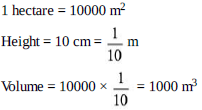QUESTION: 4

In a swimming pool measuring 90 m by 40 m, 150 men take a dip. If the average displacement ofwater by a man is 8 cubic metres, what will be rise in water level?

Solution:

Let the rise in height be = h
Then, as per the question, the volume of water should be equal in both the cases.
Now, 90 × 40 × h = 150 × 8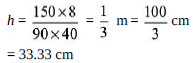QUESTION: 5

Two cones have their heights in the ratio 1 : 2 and the diameters of their bases are in the ratio 2 :1. What will be the ratio of their volumes?

Solution:

If the ratio of their diameters = 2 : 1, then the ratio of their radii will also be = 2 : 1
Let the radii of the broader cone = 2 and height be = 1
Then the radii of the smaller cone = 1 and height be = 2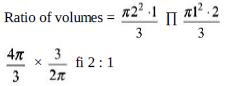QUESTION: 6

A closed wooden box measures externally 10 cm long, 8 cm broad and 6 cm high. Thickness ofwood is 0.5 cm. Find the volume of wood used.

Solution:

Volume of wood used = External volume – Outer Volume
fi (10 × 8 × 6) – (10 – 1) × (8 – 1) × (6 – 1)
fi 480 – (9 × 7 × 5) = 165 cm2

QUESTION: 7

Three cubes each of volume of 216 m3 are joined end to end. Find the surface area of the resultingfigure.

Solution:

Let one side of the cube = a
Then a3 = 216 fi a = 6 m
Area of the resultant figure
= Area of all 3 cubes – Area of covered figure
fi 216 × 3 – (4 × a2) fi 648 – 144 fi 504 m2

QUESTION: 8

The largest cone is formed at the base of a cube of side measuring 7 cm. Find the ratio of volumeof cone to cube.

Solution: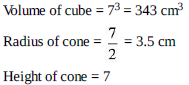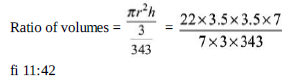QUESTION: 9

Find the radius of the circle inscribed in a triangle whose sides are 8 cm, 15 cm and 17 cm.

Solution: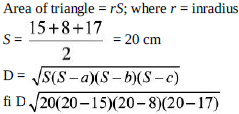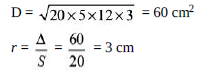QUESTION: 10

A cube whose edge is 20 cm long has circle on each of its faces painted black. What is the totalarea of the unpainted surface of the cube if the circles are of the largest area possible?

Solution:

Surface area of the cube = 6a2 = 6 × (20)2
= 2400
Area of 6 circles of radius 10 cm = 6pr2
= 6 × p × 100
= 1885.71
Remaining area = 2400 – 1884 = 514.28

QUESTION: 11

In the adjacent figure, find the area of the shaded region. (Use = 22/7)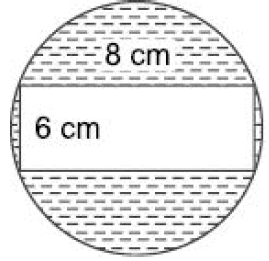Solution: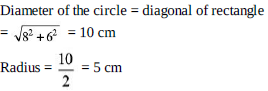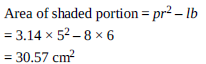QUESTION: 12

The perimeter of a sector of a circle of radius 5.7 m is 27.2 m. Find the area of the sector.

Solution:

Let the angle subtended by the sector at the centre be = q
Then,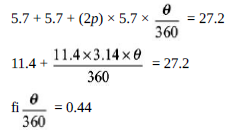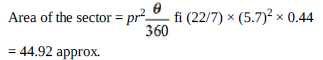QUESTION: 13

A circular tent is cylindrical to a height of 3 metres and conical above it. If its diameter is 105 mand the slant height of the conical portion is 53 m, calculate the length of the canvas 5 m wide tomake the required tent.

Solution: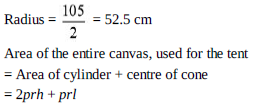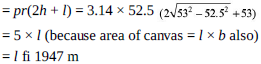QUESTION: 14

If the curved surface area of a cone is thrice that of another cone and slant height of the secondcone is thrice that of the first, find the ratio of the area of their base.

Solution: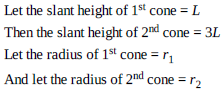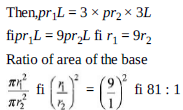QUESTION: 15

Two circles touch internally. The sum of their areas is 116p cm2 and distance between theircentres is 6 cm. Find the radii of the circles.

Solution:

Let the radius of the bigger circle = R
Let the radius of the smaller circle = r
Then as per question; R – r = 6
Solving through options; only option (a) satisfies this condition.